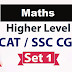## Advance Maths Questions for SSC CGL CAT

Here we are giving Advance Maths Questions for SSC CGL CAT. practice these question to crack SSC CGL and CAT exams. These questions are very useful to crack these exams. These questions cover all the important topics likes Algebra, number system, permutation & Combination, geometry, mensuration.

Q1. How many different integers can be expressed as the sum of three distinct members of the set {1, 4, 7, 10, 13, 16, 19}?
 (a) 13 (b) 16 (c) 24 (d) 30

Q2. At how many maximum distinct points, five circles in the same plane can intersect?
 (a) 12 (b) 20 (c) 24 (d) 36

Q3. Sum of 18 consecutive positive integers is a perfect square. The smallest possible value of this sum is
 (a) 169 (b) 225 (c) 289 (d) 361

Q4. A point P is randomly selected from the rectangular region with vertices (0, 0), (2, 0), (2, 1), (0, 1). What is the probability that P is closer to the origin than it is to the point (3, 1)?
 (a) 1/2 (b) 2/3 (c) 3/4 (d) 4/5

Q5. Let n be a 5-digit number, and let q and r be the quotient and the remainder, respectively, when n is divided by 100. For how many values of n is q+r divisible by 11?
 (a) 8180 (b) 8181 (c) 8182 (d) 9000

Q6. Average of the nine numbers in the set {9, 99, 999, 9999, … , 999999999} is a 9-digit number M, all of whose digits are distinct. The number M does not contain the digit
 (a) 0 (b) 2 (c) 4 (d) 6

Q7. HCF of two natural numbers is 10 and their sum is 100. How many sets of values are possible?
 (a) 10 (b) 2 (c) 4 (d) 5

Q8. (Fill in the blanks) Sum of the squares of marbles the three children have is 600, and the sum of
products taken two at a time of marbles is 150. Then, the sum of all the three numbers is…….

Q9. Samar sets his watch to the correct time at noon. At the actual time of 1:00 PM, he notices that his watch reads 12:57 and 36 seconds. Assuming that his watch loses time at a constant rate, what will be the actual time when his watch first reads 10:00 PM?
 (a) 10:22 PM and 24 seconds (b) 10:25 PM (c) 10:25:24 PM (d) None of these

Q10. J and M live 13 km apart. Yesterday, J started to ride his bicycle toward M's house. A little later, M started to ride his bicycle towards J's house. When they met, J had ridden for twice the length of time as M and at four-fifths of M's speed. How many km had M ridden when they met?
 (a) 4 (b) 8 (c) 6 (d) 5

Q11. A and B are two horses participating in a horse race with a total of six horses. How many
different arrangements of finishes are there if A always finishes before B and there is no tie in
ranking?
 (a) 720 (b) 360 (c) 120 (d) 24

Q12. In ΔABC, points P, Q, and R are the mid-points of side AB, BC, and CA, respectively. If area of ΔABC is 20 sq. units, then find the area of ΔPQR.
 (a) 10 sq. units (b) 5√3 (c) 5 sq. units (d) None of these

Q13. In a hotel, P% of total the rooms are deluxe rooms, and Q% of the total rooms are painted light blue. Which of the following best represents the minimum possible percentage of the light blue painted deluxe rooms?
 (a) P-Q (b) P-Q+100 (c) P+Q-100 (d) None of these

Q14. The numbers x and y are three-digit positive integers, and x + y is a four-digit integer. The tens digit of x equals 7 and the tens digit of y equals 5. If x < y, which of the following must be true?
I. The units digit of x + y is greater than the units digit of either x or y.
II. The tens digit of x + y equals 2.
III. The hundreds digit of y is at least 5.
 (a) II only (b) III only (c) I and II only (d) I and III only

Q15. Assume that July of a particular year has five Mondays. Which of the following will definitely occur five times in the August of the same year?
 (a) Monday (b) Tuesday (c) Wednesday (d) Thursday

Q16. The sequence S1, S2, S3, … , S10 has the property that every term beginning with the third is the sum of the previous two. That is, Sn = Sn-2 + Sn-1 for n ≥ 3.
Suppose that S9 = 110 and S7=42. What is S4?
 (a) 4 (b) 6 (c) 10 (d) 12

Q17. The angles in a particular triangle are in arithmetic progression, and the side lengths are 4, 5, x. The sum of the possible values of x equals a + b + c where a, b, and c are positive integers. What is a + b + c?
 (a) 36 (b) 38 (c) 40 (d) 42

## Solution

Dear Aspirants solve these above questions and send Ans/Solution in comment box.
We will update correct Answer & Solution Tomorrow.

Advance Maths Questions for SSC CGL CATReviewed by Super Pathshala on 11:43 Rating: 5